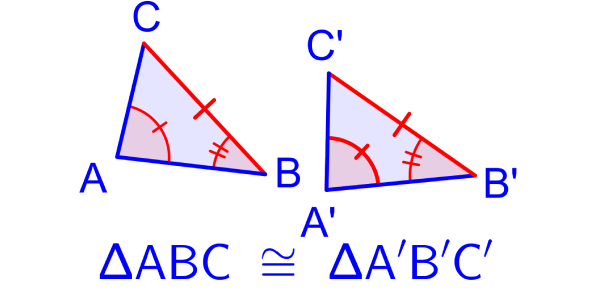# Basic Geometry Quiz For 9th Grade!

10 Questions | Attempts: 22381SettingsThis is a basic maths quiz for 9th-grade students covering the concepts of geometry. You have to solve the word problems using the mind rather than the calculator. There is a list of 10 basic questions. Read it carefully and take your time. So, let's try out the quiz. All the best!

• 1.
How many sides does the triangle have?
• A.

3

• B.

2

• C.

4

• D.

9

• E.

1

• 2.
An octagon is member of the polygons. How many sides does it have?
• A.

2

• B.

4

• C.

6

• D.

9

• E.

8

• 3.
A rectangle has a length of 6 inches and a width of 4 inches. The area is in2
• A.

24

• B.

27

• C.

26

• D.

22

• 4.
The area of a rectangle is 45 cm2. If its length is 9 cm, then its width in cm
• A.

5

• B.

10

• C.

15

• D.

None of the above

• 5.
The perimeter of a square is 24 cm. The area of the square is cm2
• A.

36

• B.

32

• C.

30

• D.

35

• 6.
How many squares with the side of 2 cm cover the surface of a rectangle with a length of 24 cm and a width of 8 cm?
• A.

48

• B.

49

• C.

47

• D.

46

• 7.
A triangle with one angle greater than 90 degrees
• A.

Equilateral triangle

• B.

Obtuse triangle

• C.

Acute triangle

• D.

Isosceles triangle

• 8.
A triangle with all three angles less than 90 degrees
• A.

Equilateral triangle

• B.

obtuse triangle

• C.

Acute triangle

• D.

Isosceles triangle

• 9.
A triangle having at least two equal sides.
• A.

acute triangle

• B.

Isosceles triangle

• C.

Equilateral triangle

• D.

Obtuse triangle

• 10.
A triangle with one angle equal to 90 degrees.
• A.

Right triangle

• B.

Equilateral triangle

• C.

Acute triangle

• D.

obtuse triangle

## Related TopicsBack to top
×

Wait!
Here's an interesting quiz for you.